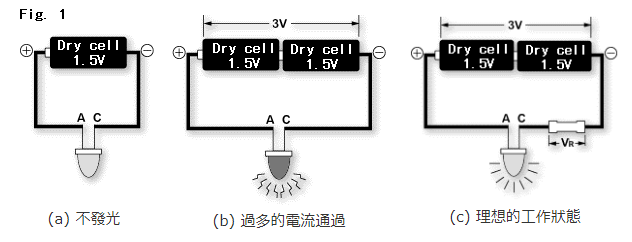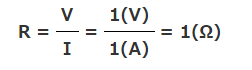# 電阻是什麼？ 基本知識

## 1. 比方說，我們想要讓發光二極體順利發光。## 2. 接下來，請各位回想一下歐姆定律。

V=R・I…(1) 其中，R為電壓V與電流I之比例常數，我們將它稱為電阻(單位為Ω)，電阻這個電子零件的功能正是如此。

## 3. 那麼，圖1-(c)的電阻值是多少？

(1)可知電阻R為...V=R・I=5(Ω)×1(A)=5(V)

VR=3(V)−2(V)=1(V)P(W)=V(V)・I(A)

1(V)・1(A)=1(W)

## 5. 串聯與並聯

(a) 串聯時的總阻值為10Ω+40Ω=50Ω

(b) 並聯時的總阻值為